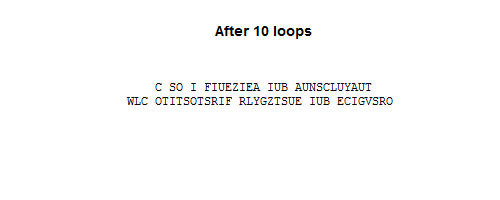Home > Misc

# Monte Carlo for substitution ciphers

The Markov chain Monte Carlo revolution by Persi Diaconis opens with an example now used as an exercise for undergraduates. The goal is to decrypt some text that's been encrypted by a simple substitution cipher. Al-Kindi was using frequency analysis to break substitution ciphers in the 9th century, so the present application of Monte Carlo is very much in the "toy problem" category, but it's a fun idea and I enjoyed seeing it in action.

We start by gathering frequencies of letter-pairs from a suitably large block of English text; I used the KJV Bible, about 4.3MB worth of text, though I haven't given much thought to how much text we actually need. With the letter-pair frequencies, we can associate a "plausibility" for each map of cipher-alphabet to real-alphabet: use the map to generate the possibly-decrypted text, then find the product of the frequencies of every letter-pair in that text. The procedure is to randomly swap pairs of letters in the map; if it improves the plausibility, then the swap is accepted, otherwise a weighted coin is flipped to decide if the swap is accepted or rejected. (The latter will usually reject the swap of letters, but the occasional acceptances allow the algorithm to escape from a local optimum.)

Using single-letter frequencies would defeat the purpose of a Monte Carlo exercise, since we may as well just order the letters by frequency. By using letter-pairs, we're effectively looking at how the letters fit together. While I haven't investigated it, I suspect that the letter-pair Monte Carlo approach will work on smaller enciphered texts than a simple ordering by single-letter frequency.

The text I enciphered and then deciphered is here; that sort of length seems to be necessary to stop the code from getting stuck in wrong local optima (though that might still happen sometimes anyway). When I started with a smaller enciphered text, I sometimes got some quite bad results, such as "D TS R ARINLRNE RIC EIPTDUIMEIO BUD SOROTSOTHRA...". Here is the trajectory of a solution that found the right answer:R code follows.

``````# Sample text: KJV Bible, http://www.gutenberg.org/cache/epub/10/pg10.txt

alphabet <- "ABCDEFGHIJKLMNOPQRSTUVWXYZ"

# Create the encrypted text:
in_file <- "tocipher.txt"
cipher_txt <- readChar(in_file, file.info(in_file)\$size)
cipher_txt <- toupper(cipher_txt)
subst_alphabet <- paste(sample(strsplit(alphabet, split="")[]), sep="", collapse="")
cipher_txt <- chartr(alphabet, subst_alphabet, cipher_txt)

# Find the letter-frequency pairs from our sample text:
in_file <- "sample.txt"
sample_txt <- readChar(in_file, file.info(in_file)\$size)
sample_txt <- toupper(sample_txt)

# Initialise the letter-pair frequencies to 1 rather than zero because later we'll be
# calculating logs of these terms.
freq_mat <- matrix(rep(1, 26^2), ncol=26, nrow=26)
for (i in 1:26) {
print(i)
for (j in 1:26) {
letter_pair <- paste(substr(alphabet, i, i), substr(alphabet, j, j), sep="")
matches <- gregexpr(letter_pair, sample_txt)[]
if (matches != -1) {
freq_mat[i, j] <- length(matches)
}
}
}
freq_mat <- freq_mat / sum(freq_mat)

# Do the Monte-Carlo!  We start by writing a function to calculate the plausibility of a given string;
# The pair_probs will be freq_mat.  The plausibility itself is a product, which becomes spectacularly
# small for long strings, so instead of calculating the product, we calculate its log.
log_plausibility <- function(text_str, pair_probs) {
abet <- "ABCDEFGHIJKLMNOPQRSTUVWXYZ"
plaus <- 0
# Remove any non-letter characters (these might mess up the regexpr):
text_str <- gsub("[^A-Z]", " ", text_str)
len_str <- nchar(text_str)
for (i in 1:(len_str - 1)) {
letter1 <- substr(text_str, i, i)
letter2 <- substr(text_str, i+1, i+1)

pos1 <- regexpr(letter1, abet)
pos2 <- regexpr(letter2, abet)

# Only look up the pair-frequency if both characters are indeed letters:
if ((pos1 > 0) & (pos2 > 0)) {
plaus <- plaus + log(pair_probs[pos1, pos2])
}
}
return(plaus)
}

new_alphabet1 <- alphabet
converged <- F
iter_ct <- 1
current_streak <- 1

orig_cipher_txt <- cipher_txt
# Strip non-letter characters from the encoded text:
cipher_txt <- gsub("[^A-Z]", " ", cipher_txt)

uncipher_txt_1 <- chartr(alphabet, new_alphabet1, cipher_txt)
plaus1 <- log_plausibility(uncipher_txt_1, freq_mat)

while(!converged) {
# Randomly sample two letters
i <- sample(1:26, 2)
old_letters <- paste(substr(new_alphabet1, i, i), substr(new_alphabet1, i, i), sep="")
new_letters <- paste(substr(new_alphabet1, i, i), substr(new_alphabet1, i, i), sep="")

new_alphabet2 <- chartr(old_letters, new_letters, new_alphabet1)
uncipher_txt_2 <- chartr(alphabet, new_alphabet2, cipher_txt)
plaus2 <- log_plausibility(uncipher_txt_2, freq_mat)

prev_text <- uncipher_txt_1

if (plaus2 > plaus1) {
# Accept the transposition of the two letters
new_alphabet1 <- new_alphabet2
plaus1 <- plaus2
uncipher_txt_1 <- uncipher_txt_2
} else {
# Flip a weighted coin to decide if we accept the letter transposition.
#
# The weighting on the coin is decided by the ratio of the plausibilities.  Since the
# log-plausibilities are very small, we might have plausibility ~ 1e-1000, say, which just
# becomes zero according to the computer.  So substract plaus1 from each log-plausibility,
# which keeps the ratios the same after exponentiating, and which ensures that the larger
# of the two plausibilities is 1.
exp_plaus1 <- 1
exp_plaus2 <- exp(plaus2 - plaus1)
if (runif(1) < exp_plaus2 / (exp_plaus1 + exp_plaus2)) {
# Accept the transposition
new_alphabet1 <- new_alphabet2
plaus1 <- plaus2
uncipher_txt_1 <- uncipher_txt_2
}
}

# Check how converged we are:
if (uncipher_txt_1 == prev_text) {
current_streak <- current_streak + 1
if (current_streak > 2000) {
converged <- T
}
} else {
current_streak <- 1
}

if (iter_ct %% 100 == 0) {
print(sprintf("%d: %s; %2.2f", iter_ct, substr(uncipher_txt_1, 1, 50), plaus1))
}

iter_ct <- iter_ct + 1
}
```
```

Home > Misc